# How to use the OR function in Excel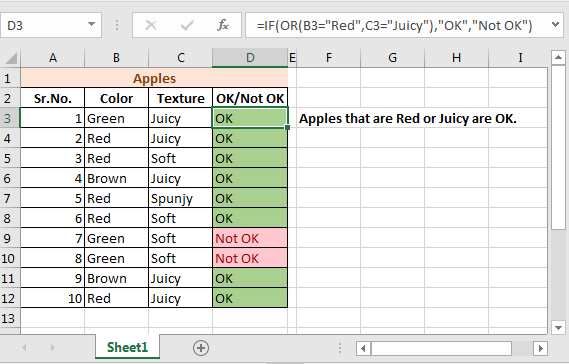In this article, we will learn How to use the OR function in Excel.

What is the OR function used for ?

OR function is logical operator. Whenever we deal with multiple values at one time, we need to check whether we need to consider different things. If you need the return value True wherever any row can have this or that. For example matching multiple employee IDs. Using OR function you can provide as many criteria you like and if any one those criteria is True. The function returns TRUE.

OR Function in Excel

OR function only returns TRUE or FALSE.

Syntax:

 =OR(logical1, [logical2], [logical3], …)

logical1 : First criteria

[logical2] :

[logical3]

Example :

All of these might be confusing to understand. Let's understand how to use the function using an example. Here we have some coloured balls and we need to test the condition to check whether the ball is valid or not.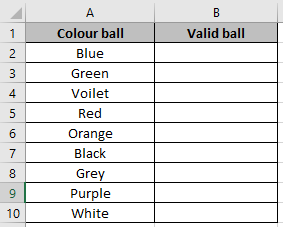Use the formula in B2 cell.

 =OR(A2="Red",A2="White")

Explanation:

Function checks either Red ball or White ball is valid for the match to be played.

So it checks the cell A2 for the same.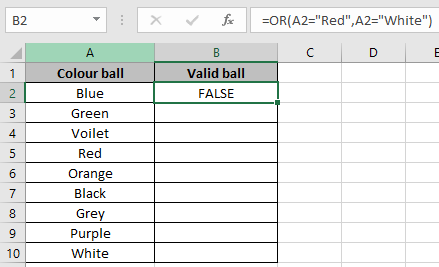As it returns False means it doesn’t have either Red or White.

Use the Ctrl + D option to check the cells corresponding to it.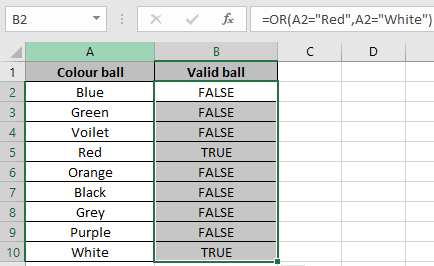As we can see in the above snapshot it returns True for the cells having Red or White coloured ball.

You can apply it to check on the range of cells to test whether any of the cell returns True or not.

Use the formula

 =OR(A2:A10="Red",A2:A10="White")

Note: Don’t forget to use Ctrl + shift + Enter to get the result. Curly brackets will be visible in the formula bar (fx) of the cell.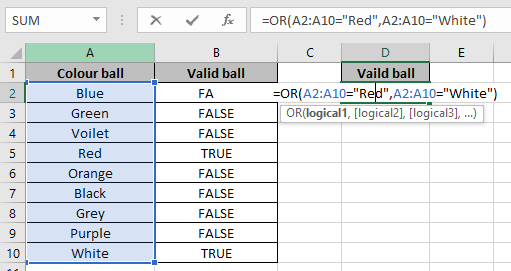Use Ctrl + Shift + Enter to see the result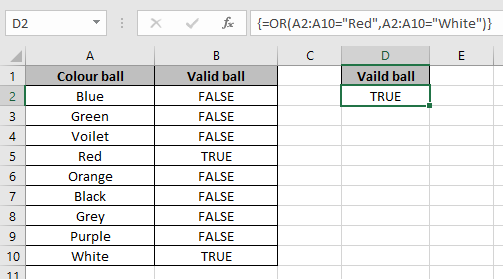It returns True. It means that A2:A10 range cells have either White or Red ball.

You can check less than or greater than condition on numbers. OR function can also be used along with many function like IF, AND and many more.

IF with OR function

We have a list of months and need to know in which quarter it lay.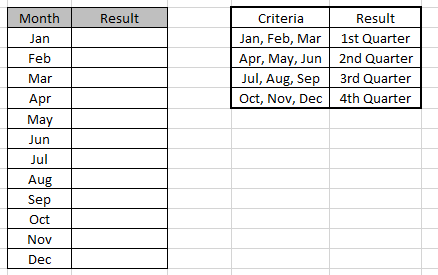Use the formula to match the quarter of the months

Formula:

 =IF(OR(A4=”Jan”,A4=”Feb”,A4=”Mar”),”1st Quarter”,IF(OR(A4=”Apr”,A4=”May”,A4=”Jun”),”2nd Quarter”,IF(OR(A4=”Jul”,A4=”Aug”,A4=”Sep”),”3rd Quarter”,IF(OR(A4=”Oct”,A4=”Nov”,A4=”Dec”),”4th Quarter”))))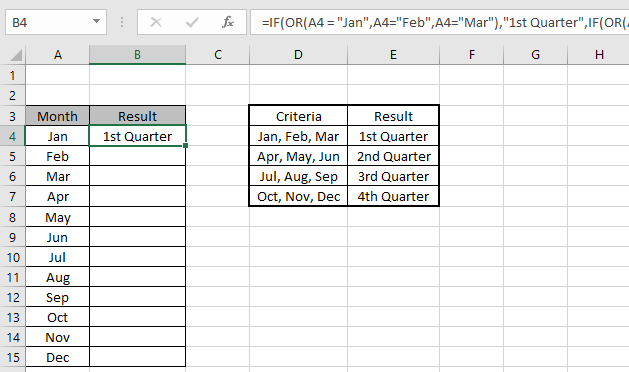Copy the formula in other cells, select the cells taking the first cell where the formula is already applied, use shortcut key Ctrl +D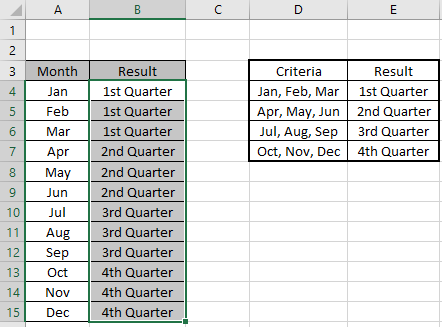We got the result.

You can also use the SUMPRODUCT function if you are comfortable with it. How to use the SUMPRODUCT function in Excel

Here are all the observational notes using the OR function in Excel
Notes :

1. Different criteria must be separated using comma ( , )
2. Operations like equals to ( = ), less than equal to ( <= ), greater than ( > ) or not equals to ( <> ) can be performed within a formula applied, with numbers only.

Hope this article about How to use the OR function in Excel is explanatory. Find more articles on checking values and related Excel formulas here. If you liked our blogs, share it with your friends on Facebook. And also you can follow us on Twitter and Facebook. We would love to hear from you, do let us know how we can improve, complement or innovate our work and make it better for you. Write to us at info@exceltip.com.

Related Articles :

How to Count Cells That Contain This Or That in Excel in Excel :To cells that contain this or that, we can use the SUMPRODUCT function. Here's how you do those calculations.

IF with OR function : Implementation of logic IF function with OR function to extract results having criteria in excel data.

IF with AND function : Implementation of logic IF function with AND function to extract results having criteria in Excel.

How to use nested IF function : nested IF function operates on data having multiple criteria. The use of repeated IF function is nested IF excel formula.

SUMIFS using AND-OR logic : Get the sum of numbers having multiple criteria applied using logic AND-OR excel function.

COUNTIFS With OR For Multiple Criteria : Count cells having multiple criteria match using the OR function. To put an OR logic in COUNTIFS function you will not need to use the OR function.

Using the IF with AND / OR Functions in Microsoft Excel : These logical functions are used to carry out multiple criteria calculations. With IF the OR and AND functions are used to include or exclude matches.

Popular Articles :

How to use the IF Function in Excel : The IF statement in Excel checks the condition and returns a specific value if the condition is TRUE or returns another specific value if FALSE.

How to use the VLOOKUP Function in Excel : This is one of the most used and popular functions of excel that is used to lookup value from different ranges and sheets.

How to use the SUMIF Function in Excel : This is another dashboard essential function. This helps you sum up values on specific conditions.

How to use the COUNTIF Function in Excel : Count values with conditions using this amazing function. You don't need to filter your data to count specific values. Countif function is essential to prepare your dashboard.

## Users are saying about us...

1.I need a formula that will check each cell in a range and only apply highlight to the entire range IF none of the cells are blank. I am having a difficult time wrapping my brain around the verbiage and logic. I read something about needing to use ctlr + shift + enter - dynamic range?? Is this true? And what is it? I have been avoiding trying to learn VBA/Macros but fear that may be my only solution if there is not a way to highlight ranges based on my criteria. Can you help? I have tried COUNTA, IFS, and a little bit of macros without much success.

•You don't need to use array formula or VBA to do this work. Use this formula in conditional formatting,
=COUNTBLANK(range)=0

and add the highlights you want to do.

If any of the cells are blank in your range than the formula will be false and nothing will happen. Else the highlights will work.

Although it does not requires Array formula, it would be greate to learn what they are. It will help you: https://www.exceltip.com/excel-array-formulas/arrays-in-excel-formula.html

Terms and Conditions of use

The applications/code on this site are distributed as is and without warranties or liability. In no event shall the owner of the copyrights, or the authors of the applications/code be liable for any loss of profit, any problems or any damage resulting from the use or evaluation of the applications/code.